# Physics - Classical Mechanics - Parallel Axis Theorem[Image 1]

## Introduction

Hey it's a me again @drifter1! Today we continue with Physics and more specifically the branch "Classical Mechanics" to get into the Parallel Axis Theorem, that's quite useful for calculating Moments of Inertia (I). So, without further ado, let's get straight into it!

## Parallel Axis Theorem

Let's consider a system of particles with a total mass M = Σmi, that's rotating about a fixed axis O. The origin of our coordinate system will be placed at the center of mass (CM) of this system of particles. So, each general point of the system has some coordinate (xi, yi). In the same way the axis O also has some coordinate (a, b). All this can be described by a diagram like this one: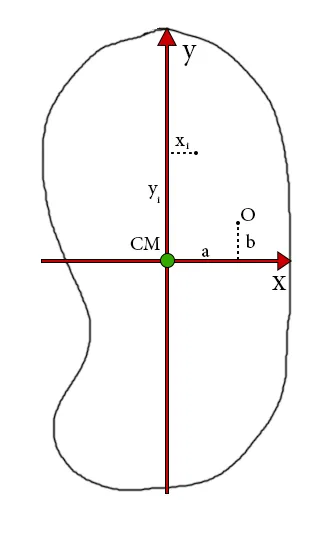Of course from the definition of the center of mass (CM) we know that:From the definition of the moment of inertia IO = Σi mi ri2O, we know that r is measured in respect to O, and not CM. But, the quantity ri2O can easily be written in respect to the center of mass as: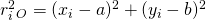Substituting this into the equation of I we get:From the two equation of the center of mass: xCM and yCM, we know that the xi and yi terms in this sum will go to zero. After that let's also split the sum into two parts: one with x's and y's and one with a's and b's. So, we end up with:The sum (xi2 + yi2) is simply equal to ri2, where ri is now being measured from the CM. So, the first sum gives us the moment of inertia for the center of mass (ICM). In the same way, we can also substitute the other quantity, cause the sum (a2 + b2) is equal to R2O, where RO is the distance from O to CM. That way the second sum turns into the simpler term MRO2. So, in the end, we finally get the Parallel Axis Theorem or Huygens-Steiner Theorem equation: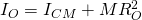To put it simply, this theorem tells us that the moment of inertia of any object about an axis is minimum when this axis is through its center of mass. For any axis parallel to that axis we have to add the term MR2O or Md2, where d is the distance of the axis of rotation (O) from the center of mass (CM). So, calculating the moment of inertia of common objects in any other axis of the object is easy, cause we just have to add the term Md2 to the moment of inertia that has already been proven for the center of mass.

To understand the Theorem even better, let's now get into an example!

## Example from physics.bu.edu

Let's consider a uniform rod of length L and mass M that's rotating about an axis through the center, perpendicular to the rod. How much is the moment of inertia for that axis?

In the previous article we said that the moment of inertia for the center of the rod, which tends to also be the center of mass, is given by: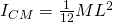Another common moment of inertia for such a rod is calculated at the end of the rod and given by: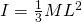Let's suppose that we don't know the moment of inertia for the center of mass (we can use it for validation), but we know the moment of inertia for the end of the rod! So, the Parallel Axis Theorem will be used for calculating ICM: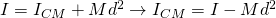The distance from the center to the end of the rod is simply L/2. By also substituting the moment of inertia that we know for the end of the rod we get:We really end up with the equation that we already know for CM!!

## RESOURCES:

### References

2. http://hyperphysics.phy-astr.gsu.edu/hbase/parax.html
3. http://physics.bu.edu/~redner/211-sp06/class-rigid-body/parallelaxis.html

### Images

1.## Final words | Next up

This is actually it for today's post! Next time we will get into Torque...which is a quite interesting topic!

See ya!Keep on drifting!

H2
H3
H4
3 columns
2 columns
1 column